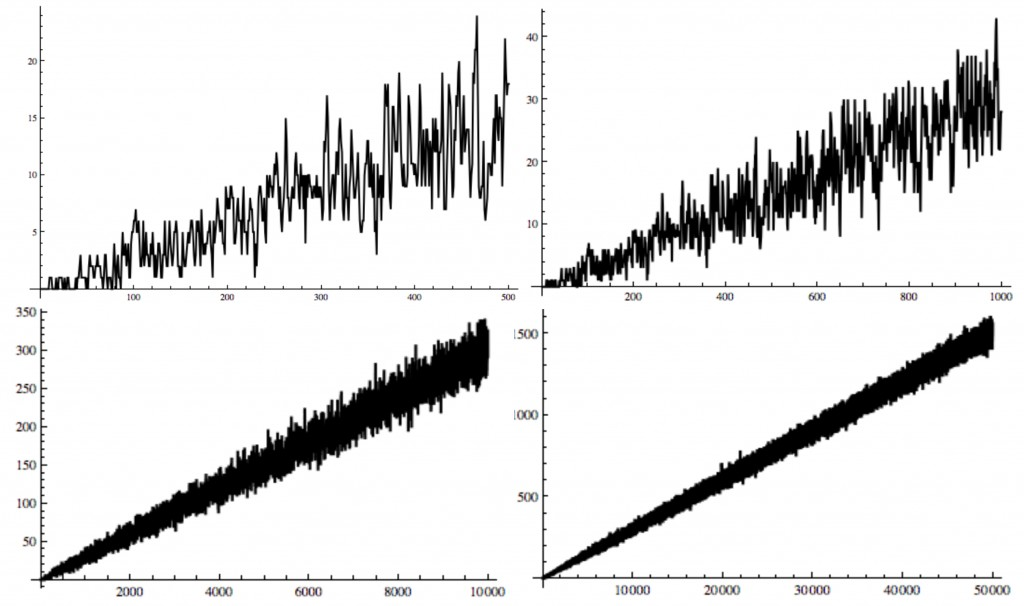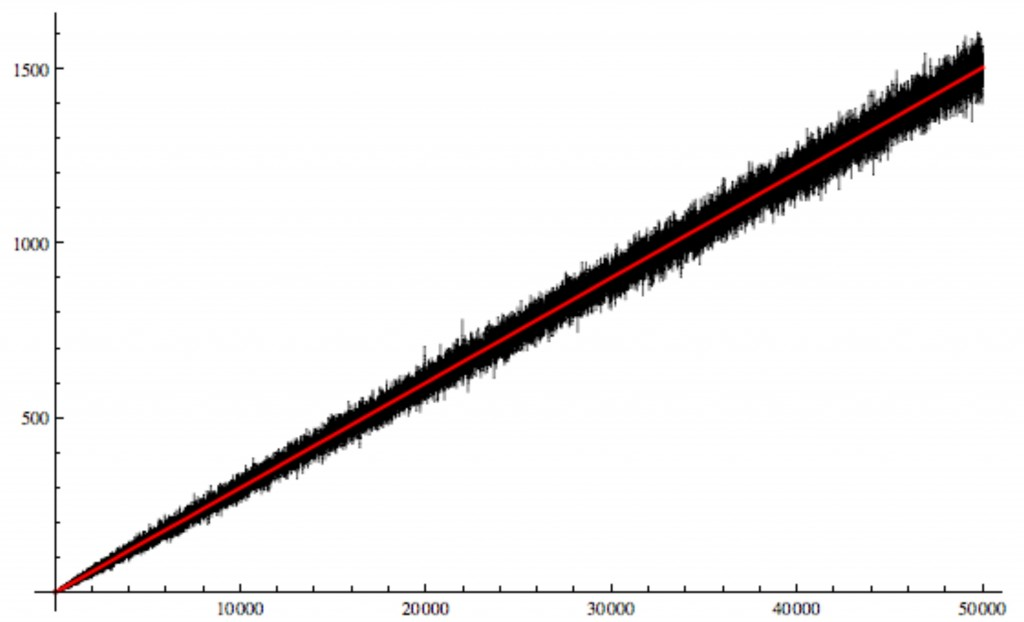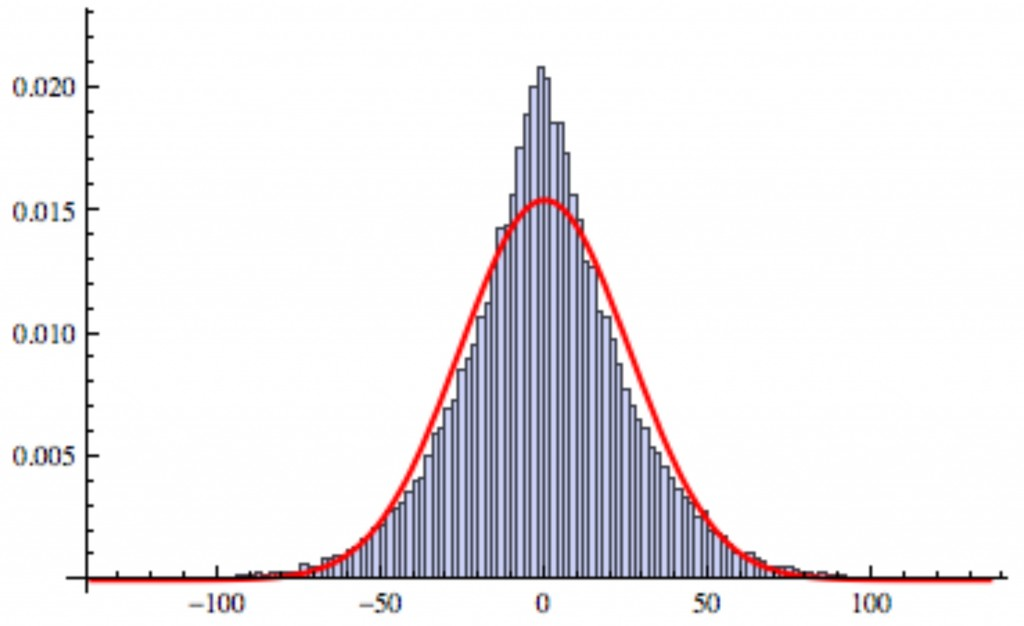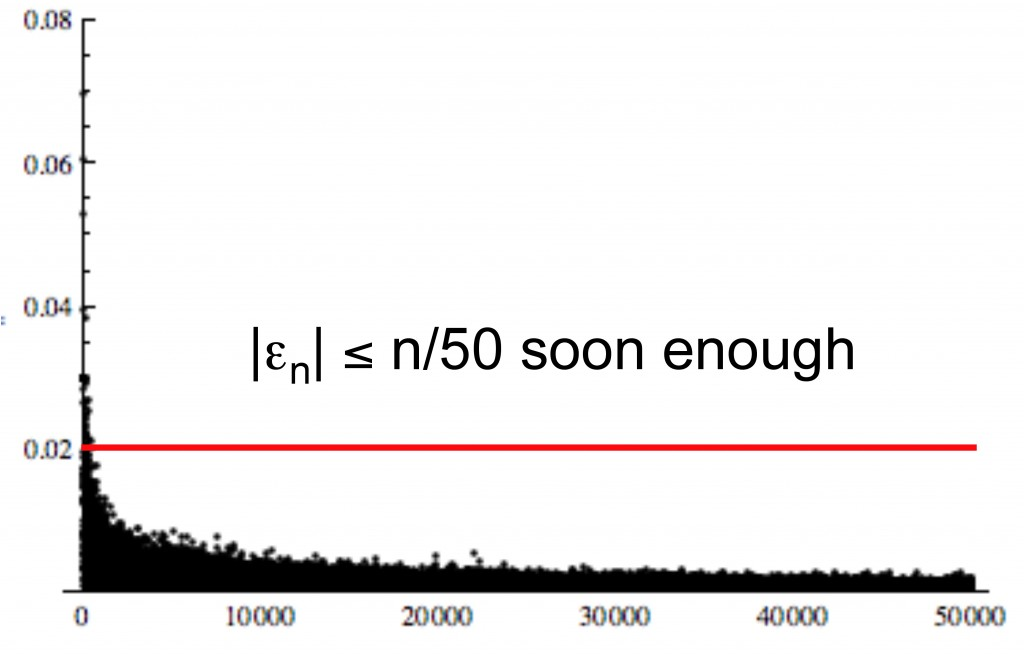# How many zeros are there in 2^n?

Posted by: Gary Ernest Davis on: April 23, 2011

An old problem asks if$2^{86}$ is the highest power of$2$ whose base$10$ representation:$2^{86}=77371252455336267181195264$ , does not contain the digit$0$.

For instance, the next$14$ powers of$2$ do indeed contain the digit$0$:$2^{87} = 1547425\textbf{0}491\textbf{0}67253436239\textbf{0}528$$2^{88} = 3\textbf{0}9485\textbf{0}\textbf{0}9821345\textbf{0}68724781\textbf{0}56$$2^{89} = 61897\textbf{0}\textbf{0}1964269\textbf{0}137449562112$$2^{90} = 123794\textbf{0}\textbf{0}3928538\textbf{0}274899124224$$2^{91} = 247588\textbf{0}\textbf{0}7857\textbf{0}76\textbf{0}549798248448$$2^{92} = 495176\textbf{0}157141521\textbf{0}99596496896$$2^{93} = 99\textbf{0}352\textbf{0}314283\textbf{0}42199192993792$$2^{94} = 198\textbf{0}7\textbf{0}4\textbf{0}628566\textbf{0}84398385987584$$2^{95} = 39614\textbf{0}81257132168796771975168$$2^{96} = 7922816251426433759354395\textbf{0}336$$2^{97} = 158456325\textbf{0}28528675187\textbf{0}879\textbf{0}\textbf{0}672$$2^{98} = 31691265\textbf{0}\textbf{0}57\textbf{0}5735\textbf{0}3741758\textbf{0}1344$$2^{99} = 6338253\textbf{0}\textbf{0}1141147\textbf{0}\textbf{0}7483516\textbf{0}2688$$2^{100} = 126765\textbf{0}6\textbf{0}\textbf{0}2282294\textbf{0}14967\textbf{0}32\textbf{0}5376$

#### How many zeros?

Instead of asking the existential question “Is there a zero in the base$10$ representation of$2^n$ for$n\geq 87$?” we can ask the more quantitative question: “How many zeros are there in the base$10$ representation of$2^n$.

Here are plots of the number of zeros in the base$10$ representation of$2^n$ for$n$ up to$100, 500, 1000, 10000, 50000$ respectively:We see, progressively, what seems to be oscillations around a straight line.

What could this straight line be?

And how large are the oscillations?

#### A straight line model

The number of digits of$2^n$ isÂ$\lfloor log_{10}(2^n)\rfloor +1$, where$\lfloor x\rfloor$ is the integer part of$x$.

Neither the first nor last digit of$2^n$ is$0$, so there are$\lfloor log_{10}(2^n)\rfloor -1$ digits of$2^n$ that can be$0$.

If we assume that all$10$ digits$0,1,\ldots 9$ are equally distributed among these$\lfloor log_{10}(2^n)\rfloor -1$ places then we expect to see about$n\frac{log_{10}(2)}{10}$ zeros in base$10$ representation of$2^n$ for large enough$n$

So we take as a linear model for the number of zeros in base$10$ representation of$2^n$ the quantity$n\frac{log_{10}(2)}{10}\approx .030103n$

To get a visual feel for how this fits let’s plot the actual number of zeros along with this linear model:#### The nature of the “error”

We now have a statistical model for the number of zeros,$Z(n)$ in the base$10$ representation of$2^n$:$Z(n)=n\frac{log_{10}(2)}{10}+\epsilon_n$

where we will refer to$\epsilon_n$ as the “error” (meaning how much$Z(n)$ differs from the linear model).

How does the error behave?

In particular, how is it distributed?

Below is a histogram of the error$\epsilon_n$ for$n\leq 50,000$, together with a normal curve of the same mean and standard deviation:We can see the the empirical distribution of the error is “peakier” than the normal distribution, and this is borne out by the kurtosis which is$3.9789 \approx 4$, substantially greater than a kurtosis of$3$ for the normal distribution.

Plotting these histograms for various values of$n$ indicates that the variance of the distribution of the errors$\epsilon_n$ varies linearly with$n$, or, what amounts to the same thing, the standard deviation varies with$\sqrt{n}$.

#### Bounding the error

What we really seek is a bound on the errors sufficient to show that$Z(n)=n\frac{log_{10}(2)}{10}+\epsilon_n>0$ for large enough$n$.

What does computation suggest?

Below is a plot of the size of the error$\vert\epsilon\vert$ divided by$n$.

It appears that$\frac{ \vert\epsilon\vert}{n}\leq \frac{1}{50}$ even for quite small$n$:If this does hold good then$Z(n)\geq n\frac{log_{10}(2)}{10} - \frac{n}{50} >0$

So now we have a more precise statement: prove that$\vert Z(n)-n\frac{log_{10}(2)}{10}\vert\leq \frac{n}{50}$ for$n\geq 200$.

Maybe techniques of higher order Fourier analysis (Higher-Order_Fourier_Tao) could be brought to bear on this problem since they are useful in proving other equidistribution theorems? Then again, just because Weyl’s polynomial equidistributionÂ  theorem can be interpreted as a higher-order Fourier analysis application may have nothing at all to do with equidistribution of digits in$2^n$.

### 6 Responses to "How many zeros are there in 2^n?"The tens digit can also never by 0. (You can reason it out or just look at the 2^n mod 100 cycle.) I don’t think it affects the slope of your model, but it would affect the intercept. Maybe that would make your histogram be better centered on the normal curve.2^22 ends in …04.@Xan: “The tens digit can also never by 0.”

2^23 = 8,388,608

Did I misinterpret what you said?I think I wrote the first and last digit, the first digit being the coefficient of 10^0.You wrote Epsilon/n <= 50 above your final figure. I think you meant to write Epsilon/n <= 1/50. Otherwise, it was an interesting read.Thanks – fixed.